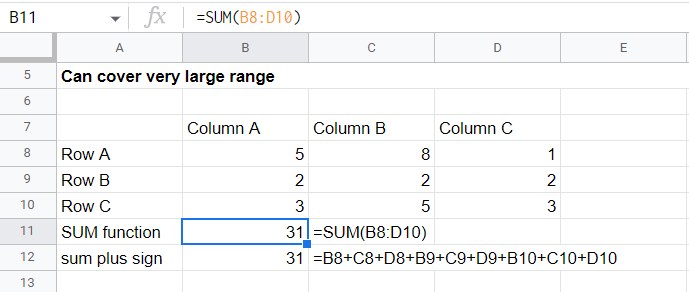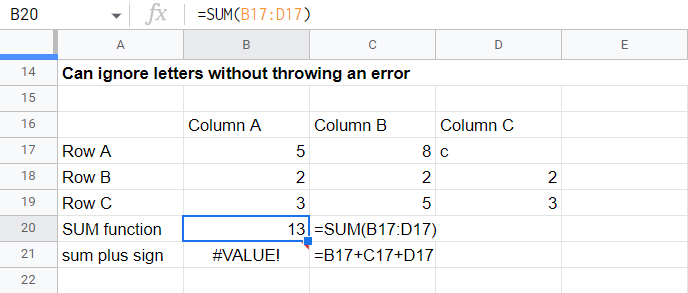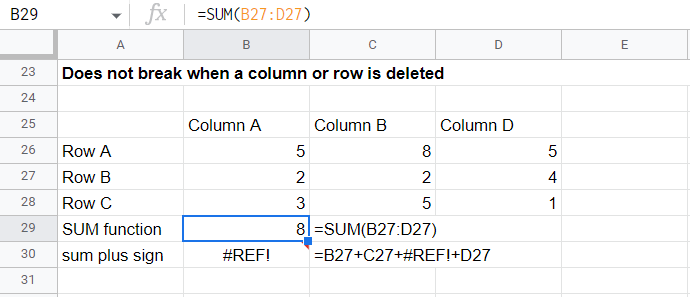# Google Sheets SUM Formula: The Ultimate Guide [2023 Update]

8 MinutesThe Google Sheets SUM function is used to add up multiple numbers in google sheets. You can enter the numbers to add manually or you can add an entire range.

SUM can save Google Sheets users a lot of time as you can easily add an entire range of numbers instead of having to enter multiple + signs between each number in a formula.

Read this tutorial to learn what you can do with the SUM function in Google Sheets.

## What is the function?

There are two ways to add values in Google Sheets. One involves using the plus sign. This is best for adding two or three numbers. However, this method can easily get taxing when you need to add several numbers, or a range of values.

If you need to add values stored in a range, you should use the SUM function. Its syntax is straightforward but you can use it in a wide variety of cases. It also has other advantages over the manual plus sign method.

#### Can cover large ranges in less timeGoogle Sheets Sum function applied to a large range. Compare with the formula using manual plus signs

#### Can ignore letters in the rangeGoogle Sheets Sum function working even with stray letters in its range.  Compare with the formula using manual plus signs

#### Does not break when a column or row is deletedGoogle Sheets Sum function working even if a column was deleted.  Compare with the formula using manual plus signs

## SUM Formula Syntax

=SUM(range)

Where

range is where the values you want to add are stored. You can specify the range as either a list of cell references or a reference to an entire range

=SUM(number1, number2, …)

Where

number1, number2, … are the numbers you want to add. You can also specify a reference to where the values you want to add are stored:

## SUM Example(s)

### Get the SUM of a column (Method 1)

#### Step 4: Press Enter

Check the screencap below:

### Get the SUM of a column (Method 2)

#### Step 1: Identify the range of the column

We want to add values in column A, so we set the range to B7:B9.

#### Step 2: Type the formula SUM(range)

The formula to type is now

=SUM(B7:B9)

### Get the SUM of a row (Method 1)

#### Step 4: Press Enter

Check the screencap below:

Google Sheets sometimes detects a pattern in your sheet and offers to extend the formula to the other cells of the row or column. You can opt to accept the suggestion or not.

### Get the SUM of a row (Method 2)

#### Step 1: Identify the range of the row

We want to add the entries of Row A, so we set the range to B18:D18.

#### Step 2: Type the formula SUM(range)

The formula to type is now

=sum(B18:D18)

#### Step 3: Select the range of the row

Hold the Ctrl key (Windows) or ⌘ key (MacOS) as you select the individual cells.

#### Step 4: Press Enter

Check the screencap below:

#### Step 1: Identify the numbers you want to add

We want to add along a diagonal of the range, which are B30, C29, and D28.

#### Step 2: Type the formula SUM(number1, number2, …)

The formula to type is now

=SUM(B30,C29,D28)

You can also hold the Ctrl key (Windows) or ⌘ key (MacOS) as you select the cells.

## Adding numbers that fulfill a certain condition

There are cases where you need to add only values that fulfill a certain condition. There are two ways of doing so in Google Sheets.

### SUM + FILTER

We combine SUM with FILTER:

=SUM(FILTER(range, condition1,  condition2, …)

Where

range is where the values you want to add are stored

condition1 and condition2 are the conditions you want to apply

#### Step 1: Identify the range of the row

We want to add all the numbers along Row 4 that are less than 4. The range is A4:C4 and the condition is A4:C4<4 .

#### Step 2: Use the formula SUM(FILTER(range,condition1,condition2,...))

The resulting formula is

=SUM(FILTER(A4:C4,A4:C4<4))